• 宽度优先搜索
2022-05-07 12:04:45

BFS即宽度优先搜索或广度优先搜索，按照层级一层层的遍历执行；
eg:
现有根结点 root [3, 9, 20, null, null, 15, 17]

1. 求二叉树距叶子节点最短路径
2. 求二叉树的最大深度

构造二叉树数据结构

public class TreeNode {
int val;
TreeNode left;
TreeNode right;

TreeNode(int val) {
this.val = val;
}
}


二叉树距叶子节点最短路径

 private static int minDepth(TreeNode root) {
if (null == root) {
return 0;
}

Queue<TreeNode> queue = new ArrayDeque<>();
int depth = 1;
while (!queue.isEmpty()) {
TreeNode treeNode = queue.poll();
if (treeNode.left == null && treeNode.right == null) {
return depth;
}

if (treeNode.left != null) {
}数据结构 深度优先
更多相关内容
• 宽度优先搜索（BFS） 1.什么时候使用BFS 1.图的遍历 -层级遍历 -由点及面（连通性） -拓扑排序 2.最短路径 -仅限简单图求最短路径 ，即图中每条边的长度都是1（一样），且没有方向。 2.解树的遍历（层级遍历） ...遍历
• 本源码是针对八数码问题的C语言实现方法，有较详细的注释。着重于广度搜索条件。大概就是这样吧。。。为啥这资源描述要这么多字。。。。
• 例8.4迷宫问题 如图所示，给出一个n*m的迷宫图和一个入口、一个出口 编写一个程序，打印从一条从迷宫入口到出口的路径。这里黑色方块的单元表示走不通（用-1表示），白色表示可以走（用0表示） ...
• 利用Java实现人工智能的八数码问题的宽度优先算法，实现对该问题的解决
• 采用宽度优先搜索算法，编程实现八数码问题的求解。初始状态和目标状态可自定；采用宽度优先搜索算法，编程实现八数码问题的求解。初始状态和目标状态可自定采用宽度优先搜索算法，编程实现八数码问题的求解。初始...
• 算法-宽度优先搜索

## 算法-宽度优先搜索

一、宽度优先搜索

广度优先或横向优先搜索，是一种图形搜索算法。简单的说，BFS是从根节点开始，沿着树的宽度遍历树的节点。如果所有节点均被访问，则算法中止。

DFS（Depth-First-Search）深度优先搜索：对每一个可能的分支路径深入到不能再深入为止，而且每个结点只能访问一次。要特别注意的是，二叉树的深度优先遍历比较特殊，可以细分为先序遍历、中序遍历、后序遍历。（它的目的是要达到被搜索结构的叶结点 ）

BFS： 广度优先搜索又叫层次遍历，从上往下对每一层依次访问，在每一层中，从左往右（也可以从右往左）访问结点，访问完一层就进入下一层，直到没有结点可以访问为止。

深度优先搜索和宽度优先搜索区别

• 二叉树的深度优先遍历的非递归的通用做法是采用栈，广度优先遍历的非递归的通用做法是采用队列
• 深度优先搜素算法：不全部保留结点，占用空间少；有回溯操作(即有入栈、出栈操作)，运行速度慢。
• 广度优先搜索算法：保留全部结点，占用空间大； 无回溯操作(即无入栈、出栈操作)，运行速度快。

二、小岛问题

class Solution {
public int numIslands(char[][] grid) {
if(grid == null || grid.length == 0) {
return 0;
}
if (grid == null || grid.length == 0) {
return 0;
}
int row = grid.length;
int column = grid.length;
boolean[][] visited = new boolean[row][column];
int number = 0;
for (int i = 0; i < row; i++) {
for (int j = 0; j < column; j++) {
if (grid[i][j] == '1' && !visited[i][j]) {
bfs(grid, i, j, visited);
number++;
}
}
}
return number;
}

public void bfs(char[][] grid, int i, int j, boolean[][] visited) {
int[] kx ={1, -1, 0, 0};
int[] ky ={0, 0, 1, -1};
visited[i][j] = true;
xQueue.offer(i);
yQueue.offer(j);
while (!xQueue.isEmpty()) {
int currentX = xQueue.poll();
int currentY = yQueue.poll();
for (int k = 0; k < 4; k++) {
int newX = currentX + kx[k];
int newY = currentY + ky[k];
if (newX >= 0 && newY >= 0 && newX < grid.length && newY < grid.length && !visited[newX][newY]) {
if (grid[newX][newY] == '1') {
xQueue.offer(newX);
yQueue.offer(newY);
visited[newX][newY] = true;
}
}
}
}
}
}


三、单词梯问题

public class Solution {
/*
* @param start: a string
* @param end: a string
* @param dict: a set of string
* @return: An integer
*/
public int ladderLength(String start, String end, Set<String> dict) {
int steps = 1;
if (dict == null) {
return 0;
}
queue.offer(start);
Set<String> duplicate = new HashSet<>();
while (!queue.isEmpty()) {
int size = queue.size();
steps++;
for (int i = 0; i < size; i++) {
String word = queue.poll();
List<String> nextWords = getNext(word, dict);
for (String next: nextWords) {
if (duplicate.contains(next)) {
continue;
}
if (next.equals(end)) {
return steps;
}
queue.offer(next);
}
}
}
return -1;
}

public List<String> getNext(String word, Set<String> dict) {
List<String> next = new ArrayList<>();
for (char i = 'a'; i <= 'z'; i++) {
for (int j = 0; j < word.length(); j++) {
String potentialNext = changedWord(word, i, j);
if (dict.contains(potentialNext)) {
}
}
}
return next;
}

public String changedWord(String word, char c, int i) {
char[] words = word.toCharArray();
words[i] = c;
return new String(words);
}

}

展开全文算法 leetcode 广度优先
• 深度优先搜索（DFS） 宽度优先搜索（BFS） c语言解题 相关例题 部分和问题 Lake Counting问题 迷宫的最短路径

## 深度优先搜索（DFS）

#### 1.定义

从某个状态开始，不断地转移状态直到无法转移，然后回退到前一步状态，继续转移到其他状态，如此不断重复，直到找到最终的解。
根据深度优先搜索的特点，采用递归函数实现比较简单。
深度优先搜索（隐式地）利用了栈进行计算。

#### 2.状态转移图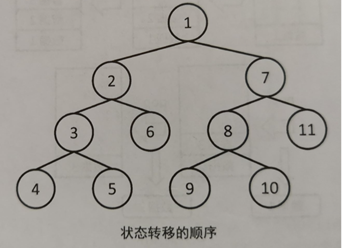#### 3.例题

##### 3.1 部分和问题

问题描述
给定正整数a1，a2，…，an，判断是否可以从中选出若干数，使他们的和恰好为k。
限制条件

• 1<=n<=20
• -108<=ai<=108
• -108<=k<=108

样例一
输入：n=4,k=13,a={1,2,4,7}
输出：Yes
解释：13=2+4+7
样例二
输入：n=4,k=15,a={1,2,4,7}
输出：No
分析
从a1开始按顺序决定每个数加或不加，在全部n个数都决定后再判断它们的和是不是k即可。因为状态数是2n，所以复杂度为O(2n)。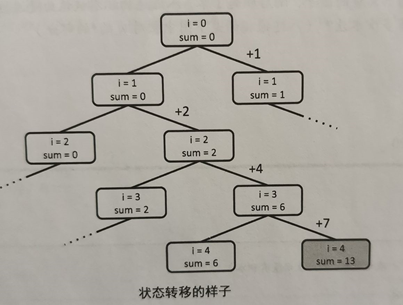代码

//DFS
#include<stdio.h>
#define MAX_N 20
int a[MAX_N]; //存储要求和的数据
int n,k;      //n代表数据个数，k代表要求出的和

//已经从前i项得到了和sum，然后对于i项之后的进行分支
int dfs(int i, int sum){
//如果前n项的都经过计算了，如何sum与k相等，返回1，否则返回0
if(i == n) return sum==k?1:0;
//不加上a[i]的情况
if(dfs(i+1, sum)) return 1;
//加上a[i]的情况
if(dfs(i+1, sum+a[i])) return 1;
}

int main(){
scanf("%d %d",&n,&k);
for(int i=0; i<n; i++) scanf("%d",&a[i]);
if(dfs(0,0)){
printf("Yes\n");
}else{
printf("No");
}
}

##### 3.2 Lake Counting

问题描述
有一个大小为N✖N的园子，雨后积起了水。八连通的积水被认为是连接在一起的。请求出园子里总共有多少个水洼？（八连通指的是下图中相对w的*部分）
限制条件

• N，M<=100

样例
输入：N=10，M=12

//园子如下图（'w'表示积水，'.'表示没有积水）
w........ww.
.www.....www
....ww...ww.
.........ww.
.........w..
..w......w..
.w.w.....ww.
w.w.w.....w.
.w.w......w.
..w.......w.


输出：3
分析
从任意的w开始，不停地把邻接的部分用’.‘代替。1次DFS后与初始的这个w所连接的所有w就都被替换成了’.’，因此直到图中不存在w为止，总共进行DFS的次数就是答案。
代码

#include<stdio.h>
#define MAX_N 100
#define MAX_M 100
int N,M;
char field[MAX_N][MAX_M];  //园子

//现在位置[x,y]
void dfs(int x,int y){
//将现在所在位置替换为.
field[x][y] = '.';

//循环遍历移动的八个方向
for(int dx = -1; dx <= 1; dx++){
for(int dy = -1; dy <= 1; dy++){
//向x方向移动dx,向y方向移动dy,移动结果为(dx,dy)
int nx = x + dx, ny = y + dy;
//判断(nx,ny)是不是在园子内,以及是否有积水
if(0<=nx && nx<=N && 0<=ny && ny<=M && field[nx][ny]=='w') dfs(nx,ny);
}
}
return ;
}

int main(){
//输入
scanf("%d %d",&N,&M);
getchar();
for(int i=0; i<N; i++){
for(int j=0; j<M; j++){
scanf("%c",&field[i][j]);
}
//将第一个for循环后面的换行符去掉，否则scanf默认读取换行符
getchar();
}
//需要DFS的次数
int res = 0;
//开始dfs
for(int i=0; i<N; i++){
for(int j=0; j<M; j++){
if(field[i][j] == 'w'){
//从有w的地方开始dfs
dfs(i,j);
res++;
}
}
}
printf("%d\n",res);
return 0;
}


## 宽度优先搜索（BFS）

#### 1.定义

与深度优先搜索不同之处在于搜索的顺序，宽度优先搜索总是先搜索距离初始状态近的状态。也就是说，它是按照开始状态→只需1次转移就可以到达的所有状态→只需2次就可以到达的所有状态→…这样的顺序进行搜索。对于同一个状态，宽度优先搜索只经过一次，因此时间复杂度为O（状态数✖转移的方式）。
宽度优先搜索利用了队列。搜索时首先将初始状态添加到队列里，此后从队列的最前端不断取出状态，把从该状态可以转换到的状态中尚未访问过的部分加入队列，如此反复，直至队列被取空或找到了问题的解。

#### 2.状态转移图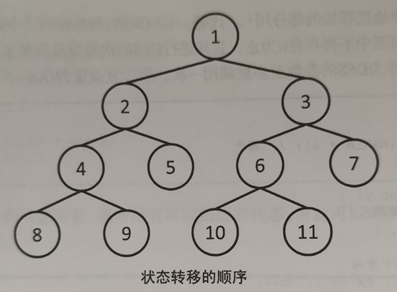#### 3.例题

##### 3.1 迷宫的最短路径

问题描述
给定一个大小为N*M的迷宫。迷宫由通道和墙壁组成，每一步可以向邻接的上下左右四格的通道移动。请求出从起点到终点所需的最小步数（起点一定可以到达终点）。
限制条件
N，M<=100
样例
输入：N=10，M=10

//迷宫如下图所示。'#','.','S','G'分别表示墙壁，通道，起点和终点。
#S######.#
......#..#
.#.##.##.#
.#........
##.##.####
....#....#
.#######.#
....#.....
.####.###.
....#...G#


输出：22
分析
宽度优先搜索按照距离开始状态由近及远的顺序进行搜索，因此可以很容易地用来求最短路径、最少操作之类问题的答案。
首先将起点存入队列，然后从队列中取出队头元素，遍历队头元素上下左右四个方向的通道。若其不是终点，则将此点加入队列，并存储从开始到该点的距离。然后处理下一个遍历到的点…直至队列为空。
代码

#include<stdio.h>
#define INF 100000000
#define MAX_N 100
#define MAX_M 100
//输入
char maze[MAX_N][MAX_M+1];  //表示迷宫的字符串的数组
int N,M;
int sx,sy;  //起点坐标
int gx,gy;  //终点坐标
int d[MAX_N][MAX_M];   //到各个位置的最短距离的数组

//队列存储结构
int queue_x[MAX_N*MAX_M];  //存储进入队列的横坐标
int queue_y[MAX_N*MAX_M];  //储进入队列的纵坐标
int front,rear;

//将坐标(x,y)加入队列queue_x,queue_y
void enQueue(int x, int y){
queue_x[rear++] = x;
queue_y[rear++] = y;
}

//将横坐标为x的移出queue_x队列
int deQueue_x(){
return queue_x[front++];
}

//将纵坐标为y的移出queue_y队列
int deQueue_y(){
return queue_y[front++];
}

//求从{sx,sy}到{gx,gy}的最短路径
//如果无法到达，则是INF
void bfs(){
//初始化
front = 0;
rear = 0;

//当前遍历到的坐标
int current_x,current_y;

//把所有的位置都初始化为INF
for(int i=0; i<N; i++)
for(int j=0; j<M; j++)
d[i][j] = INF;

//将起点加入队列，并把这一地点的距离设置为0
enQueue(sx,sy);
d[sx][sy] = 0;

//不断循环， 直到队列的长度为0
while(maze[current_x=deQueue_x()][current_y=deQueue_y()] != 'G'){
//遍历左右两面
for(int dx=-1,dy=0; dx<=1; dx+=2){
int x = current_x + dx;
int y = current_y + dy;
if(x>=0 && x<N && y>=0 && y<M){  //判断未出界
if((maze[x][y]=='G' || maze[x][y]=='.') && d[x][y]==INF){
d[x][y] = d[current_x][current_y] + 1;
enQueue(x,y);
}
}
}
//遍历上下两面
for(int dy=-1,dx=0; dy<=1; dy+=2){
int x = current_x + dx;
int y = current_y + dy;
if(x>=0 && x<N && y>=0 && y<M){   //判断未出界
if((maze[x][y]=='G' || maze[x][y]=='.') && d[x][y]==INF){
d[x][y] = d[current_x][current_y] + 1;
enQueue(x,y);
}
}
}
}
}

int main(){
scanf("%d %d",&N,&M);
getchar();
for(int i=0; i<N; i++){
for(int j=0; j<M; j++){
scanf("%c",&maze[i][j]);
//确定起点坐标
if(maze[i][j] == 'S'){
sx = i;
sy = j;
}
//确定终点坐标
if(maze[i][j] == 'G'){
gx = i;
gy = j;
}
}
getchar();
}
bfs();
//输出最终结果
printf("%d\n",d[gx][gy]);
return 0;
}

展开全文深度优先 c语言
• 易语言宽度优先搜索算法源码,宽度优先搜索算法,queue_init,queue_empty,queue_push,queue_front,queue_pop,maze
• 宽度优先搜索（BFS）与双向广搜

百度百科的官方解释：宽度优先搜索算法（又称广度优先搜索）是最简便的图的搜索算法之一，这一算法也是很多重要的图的算法的原型。Dijkstra单源最短路径算法和Prim最小生成树算法都采用了和宽度优先搜索类似的思想。其别名又叫BFS，属于一种盲目搜寻法，目的是系统地展开并检查图中的所有节点，以找寻结果。换句话说，它并不考虑结果的可能位置，彻底地搜索整张图，直到找到结果为止。

这是一个很难硬说就能理解的知识点，但是理解了就会觉得很简单（事实上所有知识点都是这样），配图理解BFS。

假设有这么一个图，1是起点，5是终点。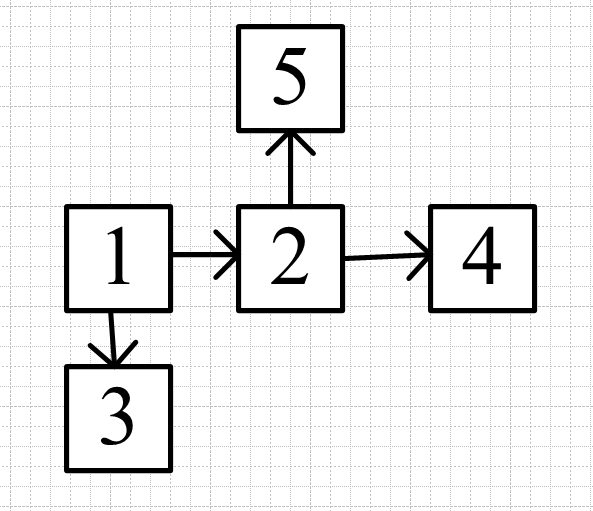遍历时将1首先加入队列（队列就是模拟BFS用的，栈是模拟DFS用的，应用的是队列先进先出和栈先进后出的特性）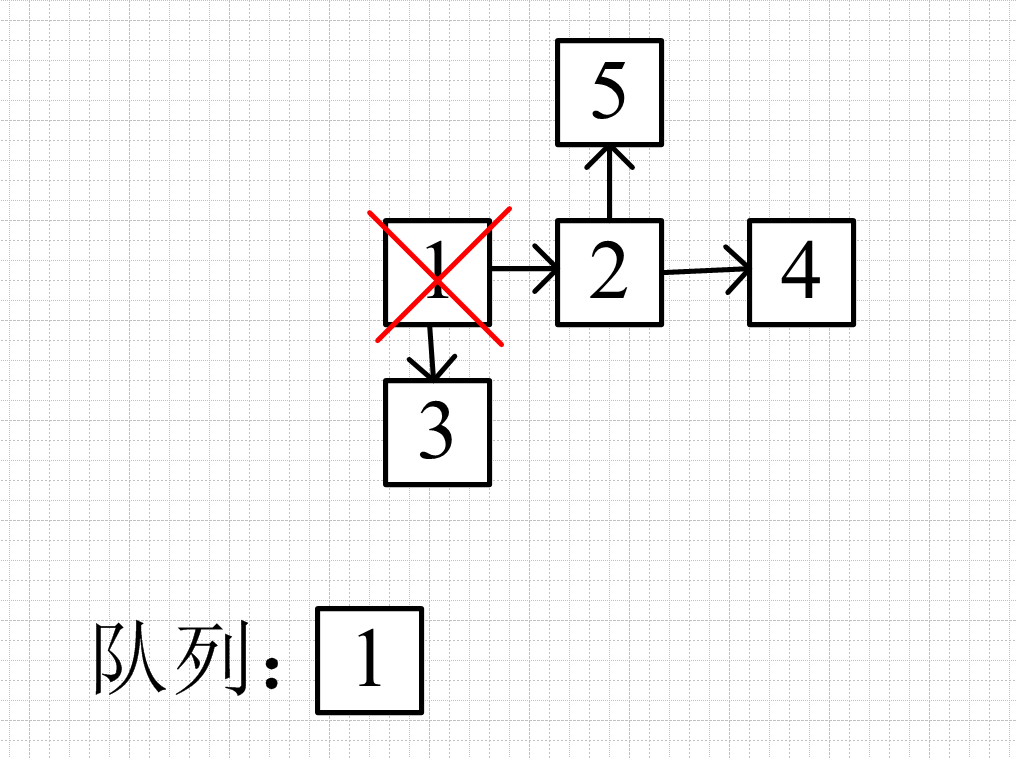然后应用一个循环模板

while(队列不为空){
弹出队头
遍历队头接下来的节点
将满足的节点加入队列
}

模拟while过程，将1弹出队列，遍历1相连的节点，将满足条件的加入队列（这里不设条件）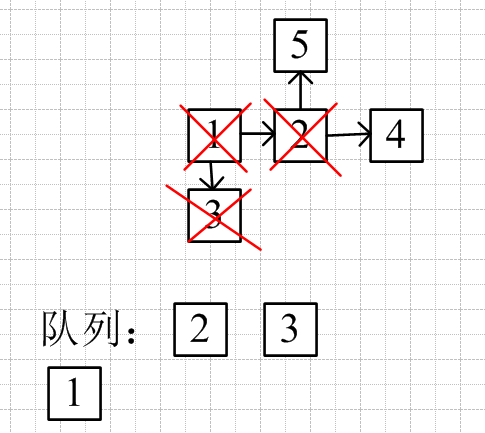弹出队头节点3，遍历与3相连的节点，与3连接的节点进队列（这里没有与3相连的），一直是从2的左边进入（即队尾进入），所以无论如何下一次都是先遍历与2相连的节点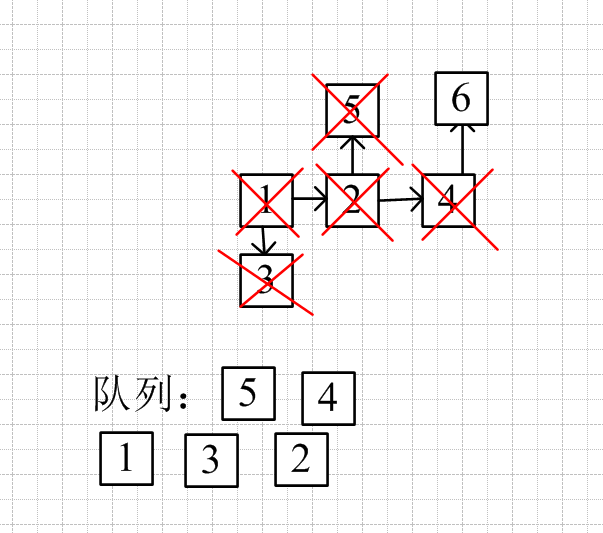比如在节点4后有一个相连的6，弹出4，加入6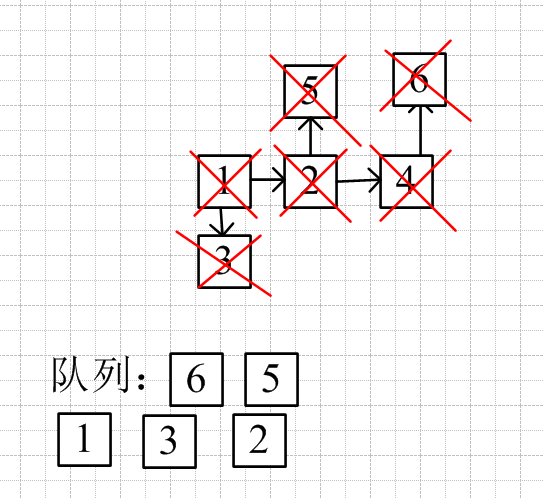但下一次的队头就是5了，而不是加入的6

这就是bfs，所有起点同步进行（类似同心圆向外扩散）

这里就会出现一个问题：如果每一个入队列的节点遍历之后都能带来接下来四个满足条件的节点，那么每一次遍历，队列就会扩大四倍，有一个比较简单的方法是引用双向广搜

双向广搜：起点终点同时加入队列，两边同时遍历，当两个节点的下一步有相交的节点，就是最短路径或者最短时间。

优化的部分可以看此图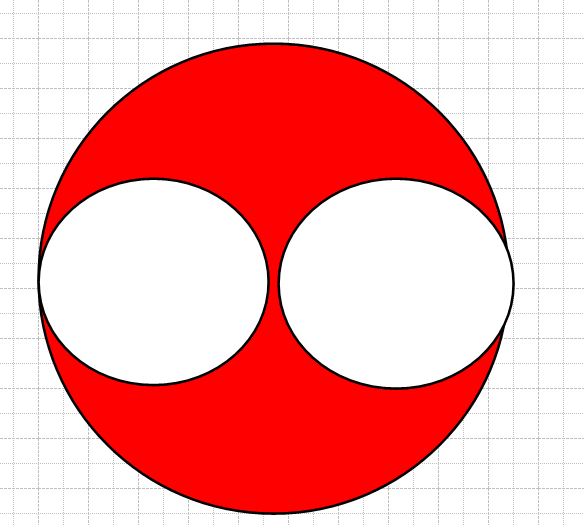红色部分是单向搜索比双向搜索多出来的部分，优化空间很大，同时也优化了时间

代码模板可变性很高，下面的看个乐就行了（毕竟bfs还是用处挺广泛的）

如果用queue模板的话记住front才是队头，如果遍历是用back就错了，因为pop弹出的front

#include "iostream"
#include "queue"
using namespace std;

struct Node{
int x;
int y;
}startNode,endNode;

int main(){
queue<Node>bfs;
bfs.push(startNode);
while(!bfs.empty()){
Node tempNode=bfs.front();
int x=tempNode.x;
int y=tempNode.y;
bfs.pop();

Node nextNode;
if(check(x+1,y)){//check是当这个条件成立时的随意一个函数，如果是迷宫
nextNode.x=x+1;//只要x+1,y这个位置不是墙壁就加入队列
nextNode.y=y;
bfs.push(nextNode);
}
if(check(x-1,y)){
nextNode.x=x-1;
nextNode.y=y;
bfs.push(nextNode);
}
if(check(x,y-1)){
nextNode.x=x;
nextNode.y=y-1;
bfs.push(nextNode);
}
if(check(x,y+1)){
nextNode.x=x;
nextNode.y=y+1;
bfs.push(nextNode);
}
}
}

展开全文数据结构
• 广度优先搜索 / 宽度优先搜索 (Breadth First Search，BFS) - 层层递进 深度优先搜索方法可用于解决从迷宫起点开始寻找一条通往迷宫中目标位置最短路径的问题。广度优先搜索 / 宽度优先搜索 (Breadth First Search，...广度优先搜索 层层递进 BFS
• 广度优先搜索 - 宽度优先搜索 - 横向优先搜索 (breadth-first search，BFS) 1. 广度优先搜索 - 宽度优先搜索 - 横向优先搜索 (breadth-first search，BFS) depth-first search，DFS：深度优先搜索 breadth-first ...广度优先搜索 BFS
• 广度优先搜索，也叫宽度优先搜索，从开始状态，到第一次能到达的状态，再从第一次能到达的状态到下一个能到达的状态，直到探索所有可到达的状态，其时间复杂度为O(状态数×转移的方式)。 广度优先搜索使用了队列的...深度优先 算法 c++ 数据结构
• 使用伪代码描述的深度优先搜索和宽度优先搜索，是两个算法的模板
• NULL 博文链接：https://iluoxuan.iteye.com/blog/1936926
• Maze试验台的设计与实现（宽度优先搜索和深度优先搜索的理解） 一、 宽度优先搜索 BFS，属于一种盲目搜寻法，目的是系统地展开并检查图中的所有节点，以找寻结果。换句话说，它并不考虑结果的可能位置，彻底地搜索整...算法 python
• 文章目录一、实验内容二、深度优先搜索和广度优先搜索总结1.深度优先搜索算法2.广度优先搜索算法三、实验代码和用于测试的迷宫1.实验代码2.测试迷宫2.1 maze1.txt2.2 maze2.txt2.3 maze3.txt四、实验结果1. maze1....python 算法 人工智能
• 宽度优先搜索之习题分析一、宽度优先搜索的概念二、小岛问题（一）、题目需求（二）、解法（三）、代码分析三、单词梯（一）、题目需求（二）、解法（三）、代码分析 一、宽度优先搜索的概念 ​ 广度优先搜索（也称...字符串 队列 算法 bfs
• 文章目录宽度优先搜索能够处理的问题代码注意事项时间复杂度分析题目汇总 宽度优先搜索能够处理的问题 宽度优先搜索的对象一般是二叉树、图、矩阵，这三种其实都可以归类为图，使用宽度优先搜索的情况有以下三种： ...
• 易语言源码易语言宽度优先搜索算法源码.rar 易语言源码易语言宽度优先搜索算法源码.rar 易语言源码易语言宽度优先搜索算法源码.rar 易语言源码易语言宽度优先搜索算法源码.rar 易语言源码易语言宽度优先搜索算法...
• ## 宽度优先搜索算法解决八数码问题

万次阅读 多人点赞 2020-05-19 23:44:41
宽度优先搜索算法解决八数码问题 实验原理 1、宽度优先搜索是指在一个搜索树中，搜索以同层邻近节点依次扩展节点。这种搜索是逐层进行的，在对下一层的任一节点进行搜索之前，必须搜索完本层的所有节点。 宽度优先...队列 python 数据结构 算法
• 宽度优先搜索（ Breadth first Search，BFS）是一种典型的数据密集型课题，被广泛应用于多种图算法。 Graph500 Benchmark以BFS搜索为核心算法，已经成为评价计算机处理大数据能力的基准。神威太湖之光超级计算机从...
• 解八数码问题：任意输入两个九宫格作为初始状态和目标状态，用宽度优先搜索求解。#include#include#include#include#includeusing namespace std;class NineNode{public:int nine;//九宫格int parent;//父节点...
• 九章算法之宽度优先搜索(Breadth First Search, BFS) 多看多思考
• 广度优先搜索算法（又称宽度优先搜索）是最简便的图的搜索算法之一，这一算法也是很多重要的图的算法的原型。...